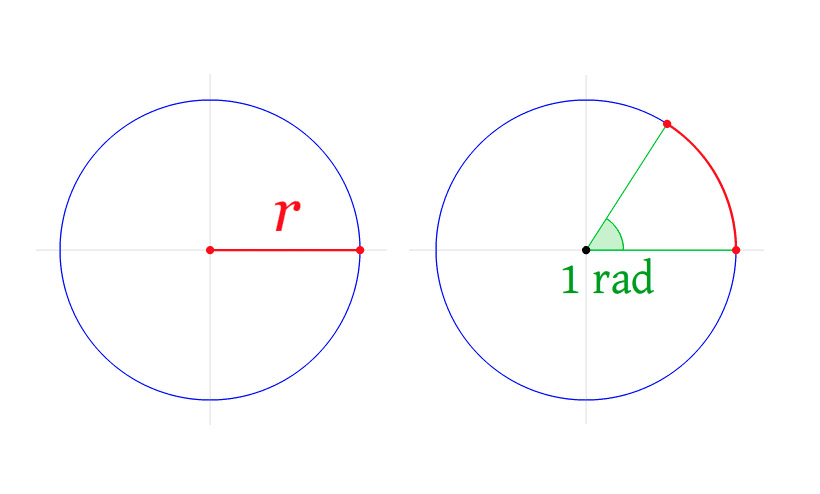### Radian

The radian is the SI (French: Système International d'Unités) unit for measuring angles. It is the standard unit of angular measure used in many areas of mathematics. Radian describes the plane angle subtended by a circular arc as the length of the arc divided by the radius of the arc. One radian is the angle subtended at the center of a circle by an arc that is equal in length to the radius of the circle.

1 rad = 180/π degrees ≈ 57.3 degreesSource: Wikipedia

A complete revolution is 2π radians. It follows that the magnitude in radians of one complete revolution (360 degrees) is the length of the entire circumference divided by the radius, or 2πr / r, or 2π. Thus 2π radians is equal to 360 degrees, meaning that one radian is equal to 180/π degrees.Source: Wikipedia